## Neural Style Transfer Implementation

Neural Style Transfer is a method of creating artistic style images using Deep Neural Networks (Convolutional Neural Networks). This algorithm was created by Gatys et al. (2015) (https://arxiv.org/abs/1508.06576). Code is adapted from Andrew Ng’s Course ‘Convolutional Neural Networks”.

## Results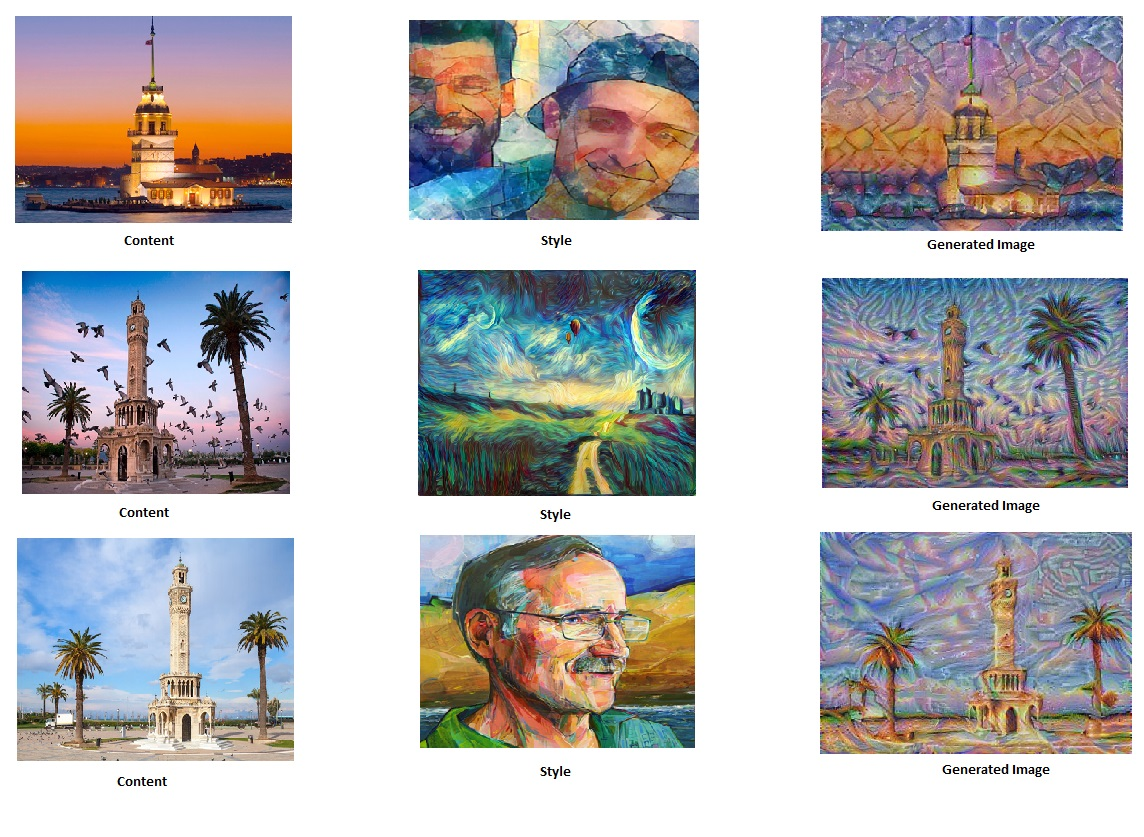## Transfer Learning

“Following the original NST paper (https://arxiv.org/abs/1508.06576), we will use the VGG network. Specifically, we’ll use VGG-19, a 19-layer version of the VGG network. This model has already been trained on the very large ImageNet database, and thus has learned to recognize a variety of low level features (at the earlier layers) and high level features (at the deeper layers)”

## Neural Style Transfer Algorithm

• Calculate the content cost function
• Calculate the style cost function
• Put it together to get $J(G) = \alpha J_{content}(C,G) + \beta J_{style}(S,G)$.
• Create an Interactive Session (tensorflow)
• Randomly initialize the image to be generated
• Build the TensorFlow graph:
• Run the content image through the VGG16 model and compute the content cost
• Run the style image through the VGG16 model and compute the style cost
• Compute the total cost
• Define the optimizer and the learning rate
• Initialize the TensorFlow graph and run it for a large number of iterations, updating the generated image at every step.

## What is Transfer Learning? Transfer Learning With Keras (ResNet50)

“Transfer learning is a machine learning technique where a model trained on one task is re-purposed on a second related task. Transfer learning is an optimization that allows rapid progress or improved performance when modeling the second task.” If training and test sets are too small to implement ML application, transfer learning is very common technique that is used for avoiding overfitting in ML application. However there are two main points that are considering: inputs of pre-trained model and transferred model must be same and  transferred model size (e.g. 1000) is smaller than pre-trained one (e.g. 1000000).  Commonly, researchers use pre-trained models  in the frameworks. For instance, Keras library added pre-trained RESNET model with trained ImageNet datasets.

With following snippet, we can use Residual  Nets (ResNet50) from Keras Library for transfer learning.

def get_model():

input_tensor = Input(shape=(224, 224, 3))  # this assumes K.image_data_format() == 'channels_last'

# Create the base pre-trained model
base_model = ResNet50(input_tensor=input_tensor,weights='imagenet',include_top=False)

# Except last layer, other layers training is frozen with 'layer.trainable=false'
for layer in base_model.layers:
layer.trainable=False

# Pooling and new softmax layers added for new application
x = base_model.output
x = GlobalAveragePooling2D(data_format='channels_last')(x)
x = Dense(num_classes, activation='softmax')(x)

# Model is updated
updatedModel = Model(base_model.input, x)

return updatedModel

## Sentiment Analysis Using LSTM and GLoVe Word Embedding

Sentiment Analysis is an analysis of the sentence, text at the document that gives us the opinion of the sentence/text. In this project, it will be implemented a model which inputs a sentence and finds the most appropriate emoji to be used with this sentence. Code is adapted from Andrew Ng’s Course ‘Sequential Models’.

Github Code: https://github.com/omerbsezer/SentimentAnalysis

## Results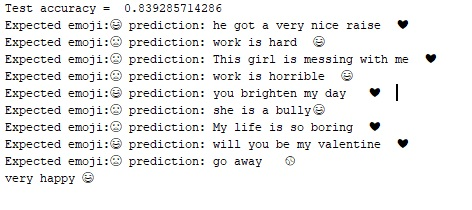## DataSet

We have a tiny dataset (X, Y) where:

• X contains 127 sentences (strings)
• Y contains a integer label between 0 and 4 corresponding to an emoji for each sentence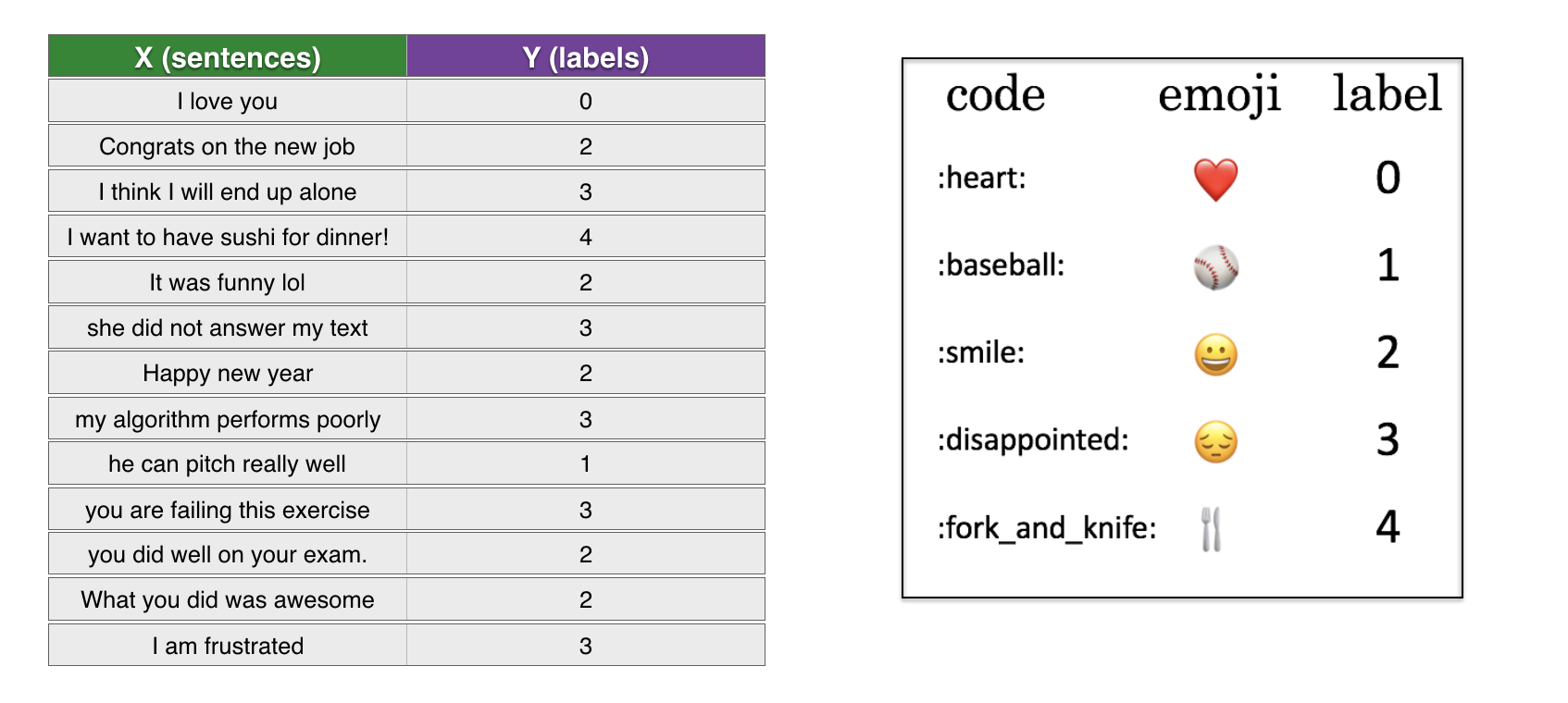## Embeddings

Glove 50 dimension, 40000 words of dictionary file is used for word embeddings. It should be downloaded from https://www.kaggle.com/watts2/glove6b50dtxt (file size = ~168MB))

• word_to_index: dictionary mapping from words to their indices in the vocabulary (400,001 words, with the valid indices ranging from 0 to 400,000)
• index_to_word: dictionary mapping from indices to their corresponding words in the vocabulary
• word_to_vec_map: dictionary mapping words to their GloVe vector representation.

## LSTM

LSTM structure is used for classification.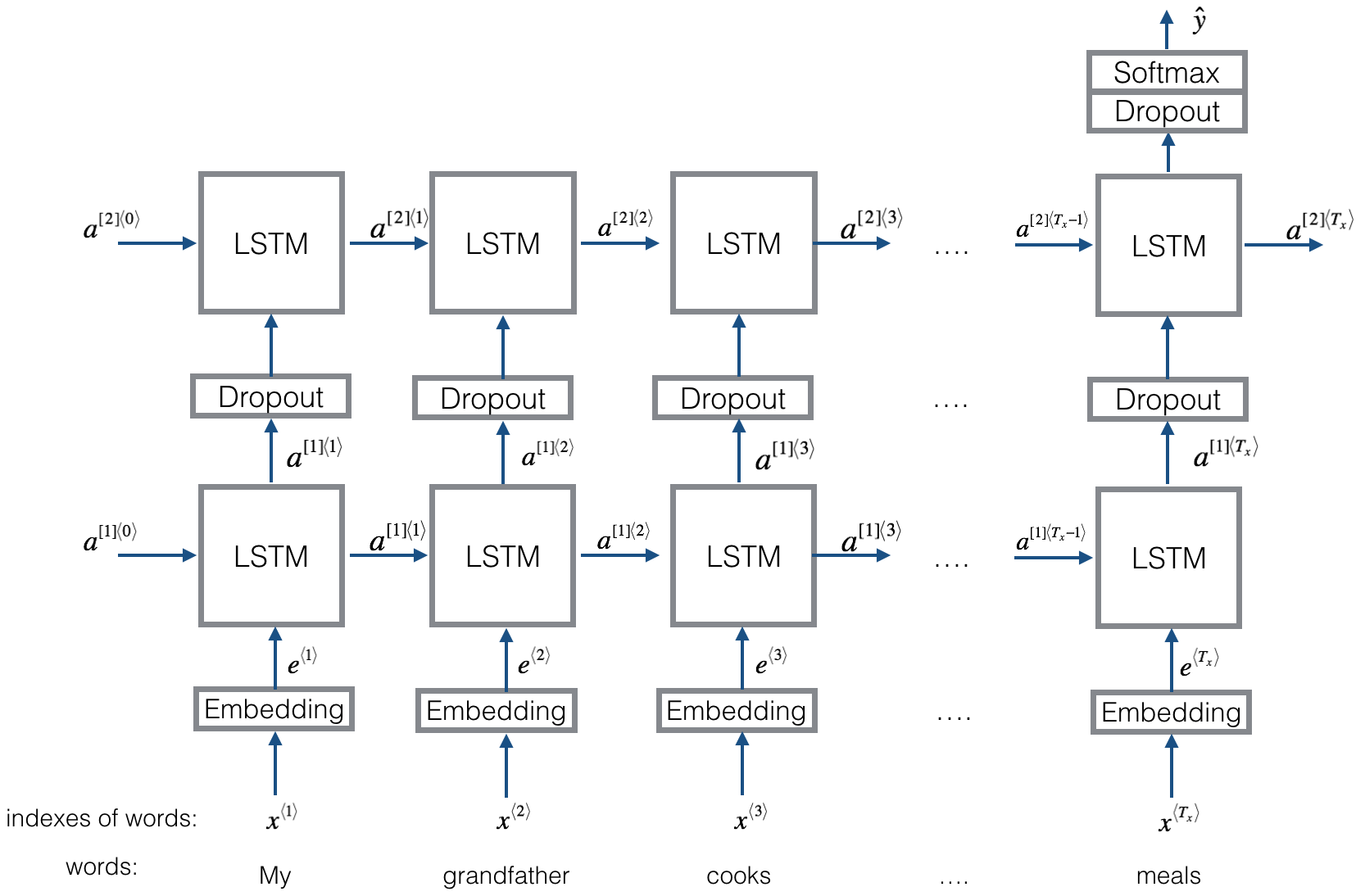Parameters: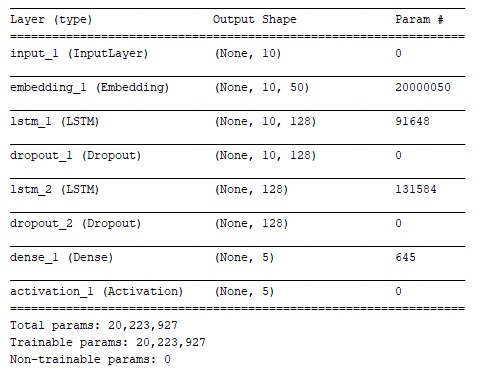## References

• Andrew Ng, Sequential Models Course, Deep Learning Specialization

## Pong Game RL application: Policy Gradient Implementation Using OpenAI Gym and Tensorflow

Policy gradient network is implemented using popular atari game, Pong Game. “Policy gradients method involves running a policy for a while, seeing what actions lead to high rewards, increasing their probability through backpropagating gradients”.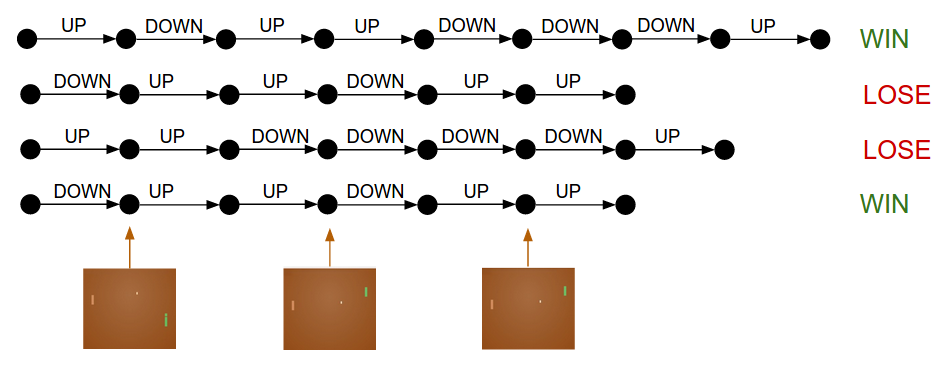If there is a large scale problems which is aimed to solve, a type of function approximator should be used. In this problem, a neural network is used as function approximator. There are too many states and/or actions to store in memory, so look up table can not be used.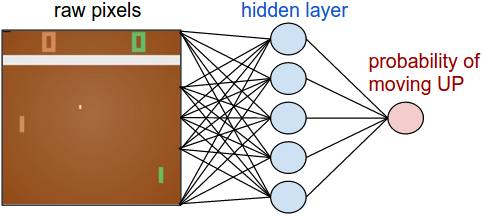Andrej Karpathy (Deep Reinforcement Learning: Pong from Pixels): http://karpathy.github.io/2016/05/31/rl/

Policy Gradient Neural Network, based on Andrej’s solution, will do:

• take in images from the game and “preprocess” them (remove color, background, etc).
• use the TF NN to compute a probability of moving up or down.
• sample from that probability distribution and tell the agent to move up or down.
• if the round is over, find whether you won or lost.
• when the episode has finished, pass the result through the backpropagation algorithm to compute the gradient for weights.
• after each episodes have finished, sum up the gradient and move the weights in the direction of the gradient.
• repeat this process until weights are tuned to the point.

### PongGame Experiment Results

After a period time, scores are getting better.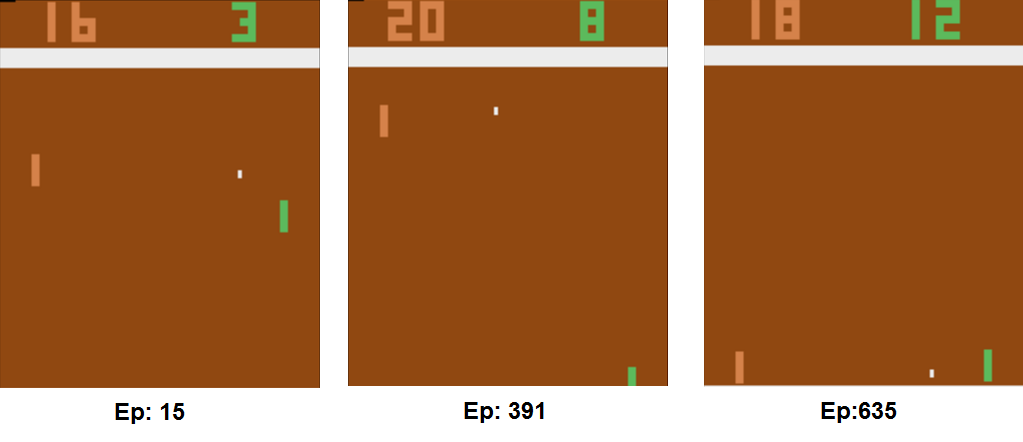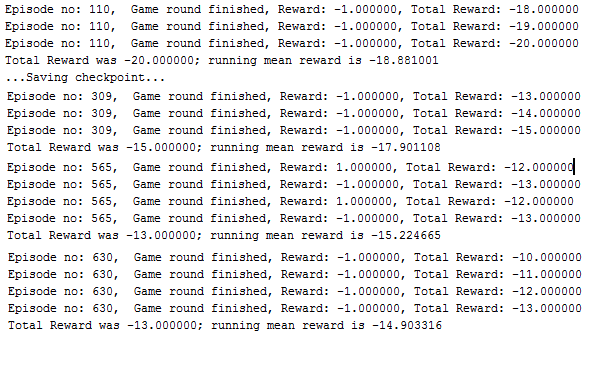After 2 days running, system is learned and starting to beat opponent. Last saved checkpoint which is learned after 2days is committed in the checkpoint folder. When starting code in your environment, if there is a checkpoint point folder, it will be loaded..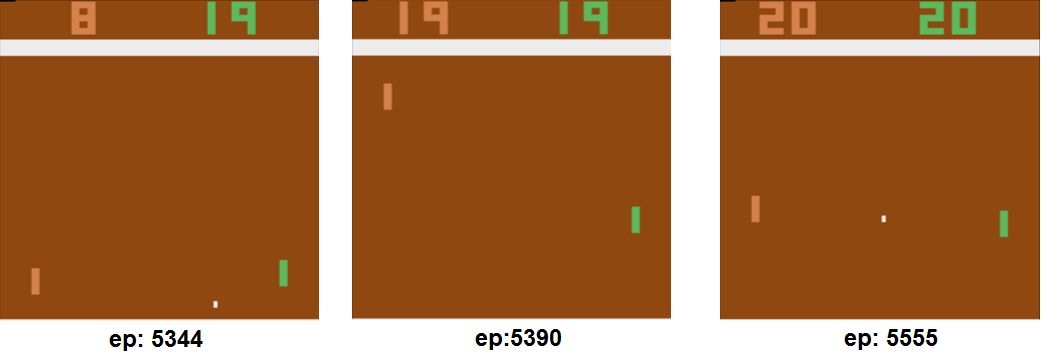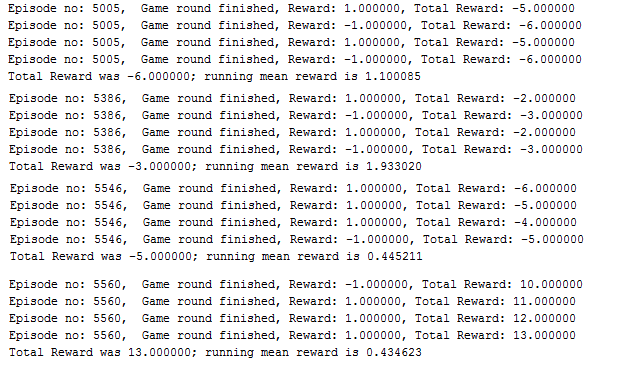### References:

Policy Gradients from David Silver: http://www0.cs.ucl.ac.uk/staff/d.silver/web/Teaching_files/pg.pdf

Pong Game Open AI Gym: https://gym.openai.com/envs/Pong-v0/

Open AI Gym: https://gym.openai.com/docs/

https://github.com/mrahtz/tensorflow-rl-pong

https://medium.com/@dhruvp/how-to-write-a-neural-network-to-play-pong-from-scratch-956b57d4f6e0

## Reinforcement Learning Application: CartPole Implementation Using QLearning

“A pole is attached by an un-actuated joint to a cart, which moves along a frictionless track. The pendulum starts upright, and the goal is to prevent it from falling over by increasing and reducing the cart’s velocity.”

Github Code: https://github.com/omerbsezer/QLearning_CartPole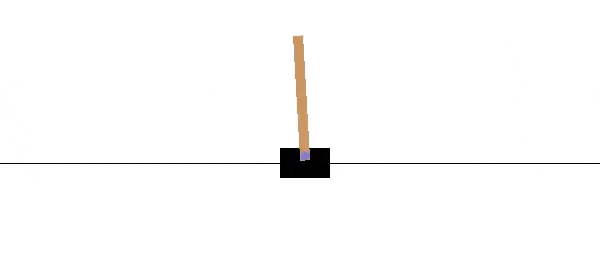### QLearning Implementation Using Gym

“QLearning is a model free reinforcement learning technique that can be used to find the optimal action selection policy using Q function without requiring a model of the environment. Q-learning eventually finds an optimal policy.” Q-learning is a specific TD (Temporal-difference) algorithm used to learn the Q-function. If there is no large scale problems, we can use look up table like in this problem.

CartPole Results: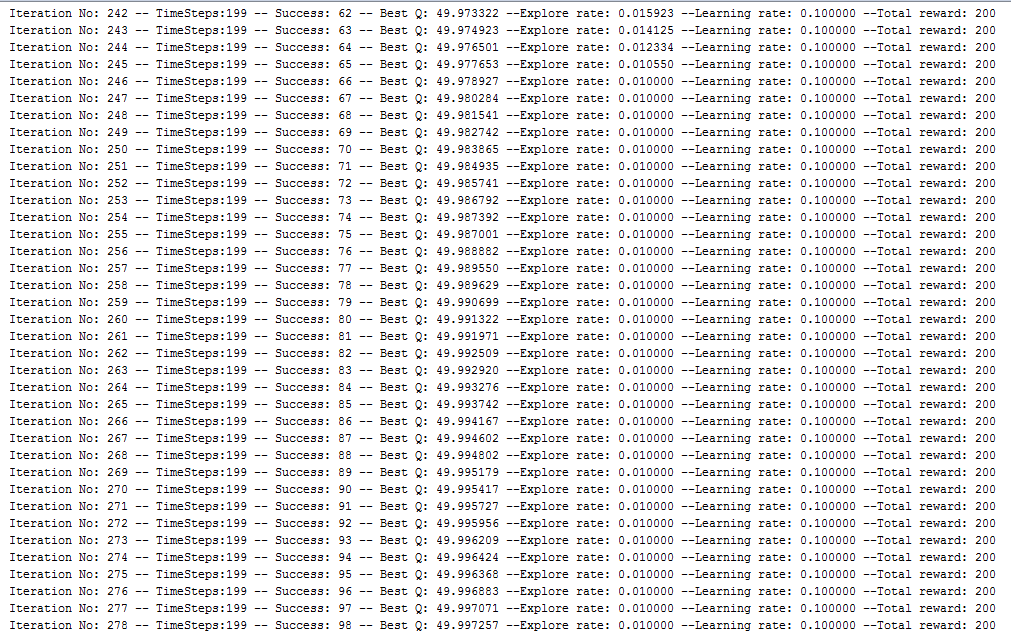Refs: QLearning: https://en.wikipedia.org/wiki/Q-learning

Cart Pole Problem: https://en.wikipedia.org/wiki/Inverted_pendulum

Cart Pole Open AI Gym: https://github.com/openai/gym/wiki/CartPole-v0

Open AI Gym: https://gym.openai.com/docs/